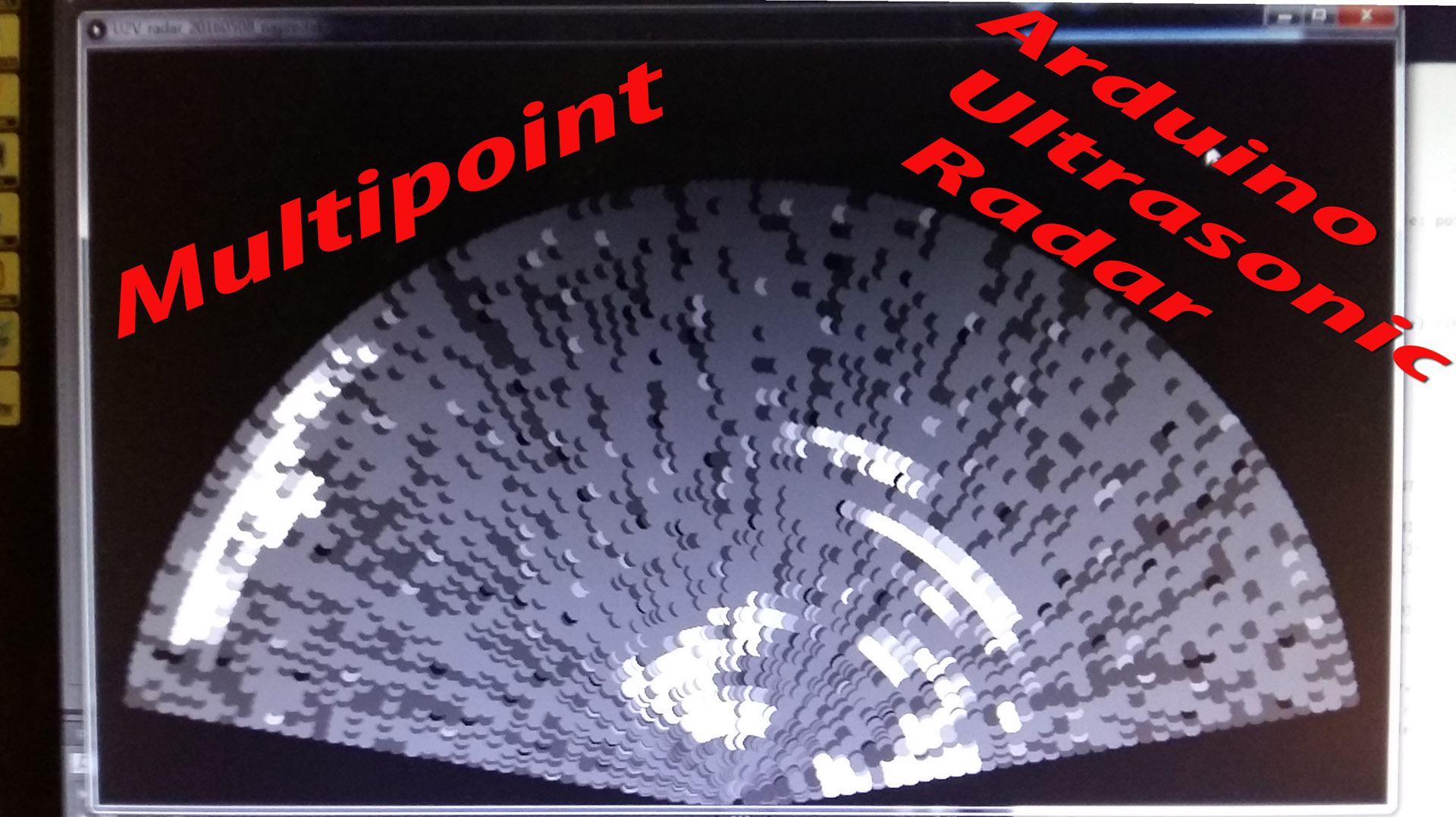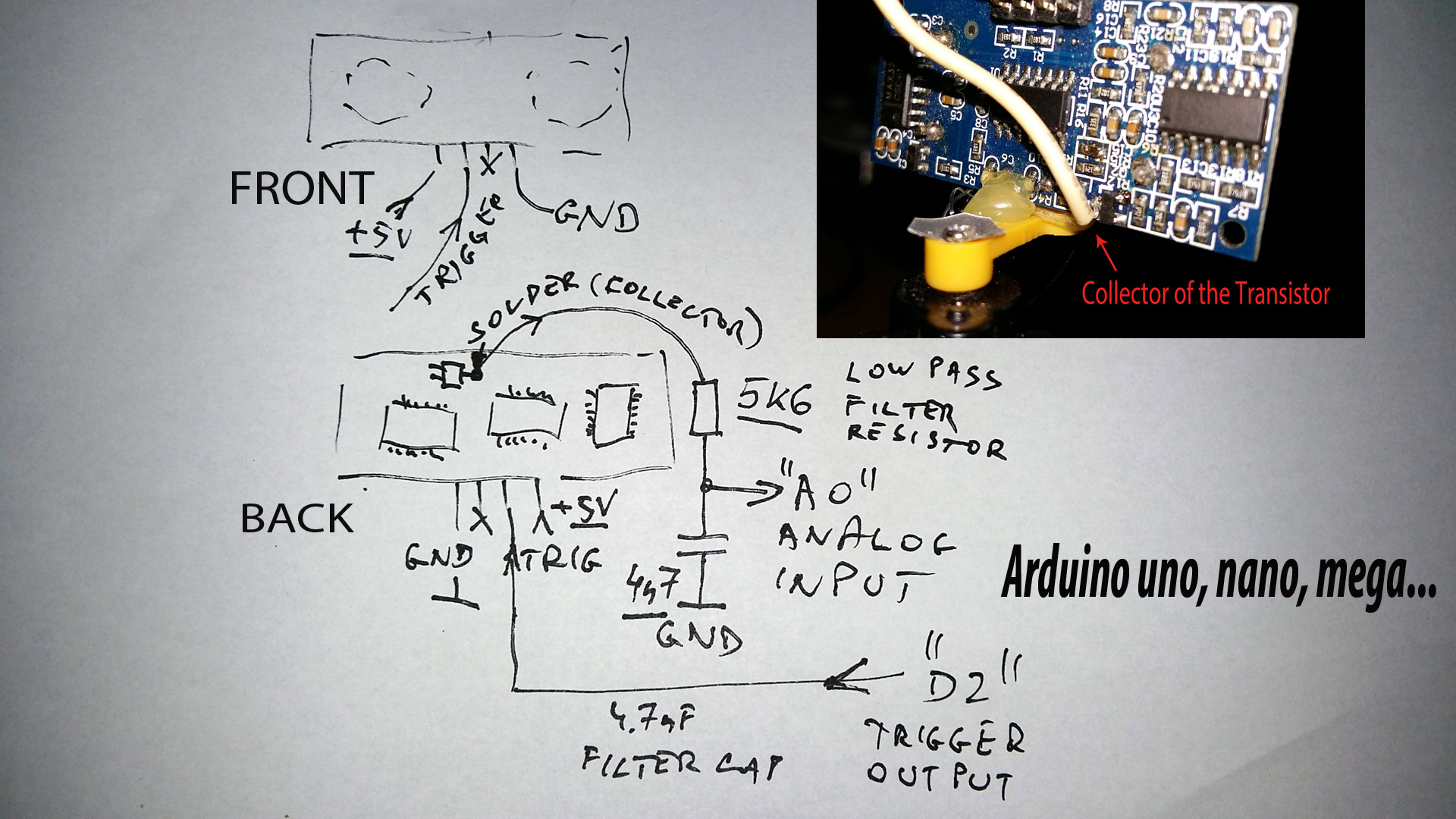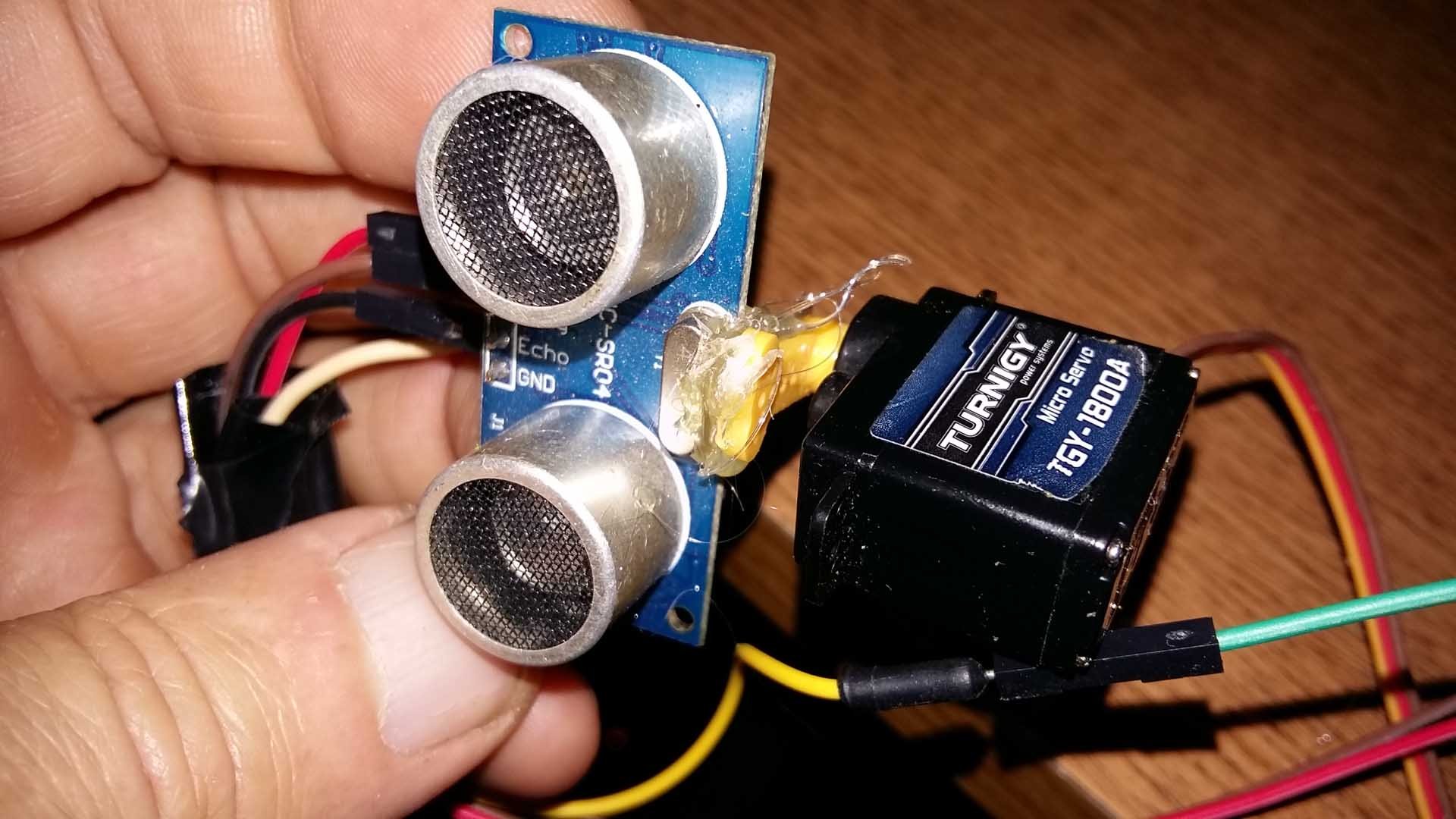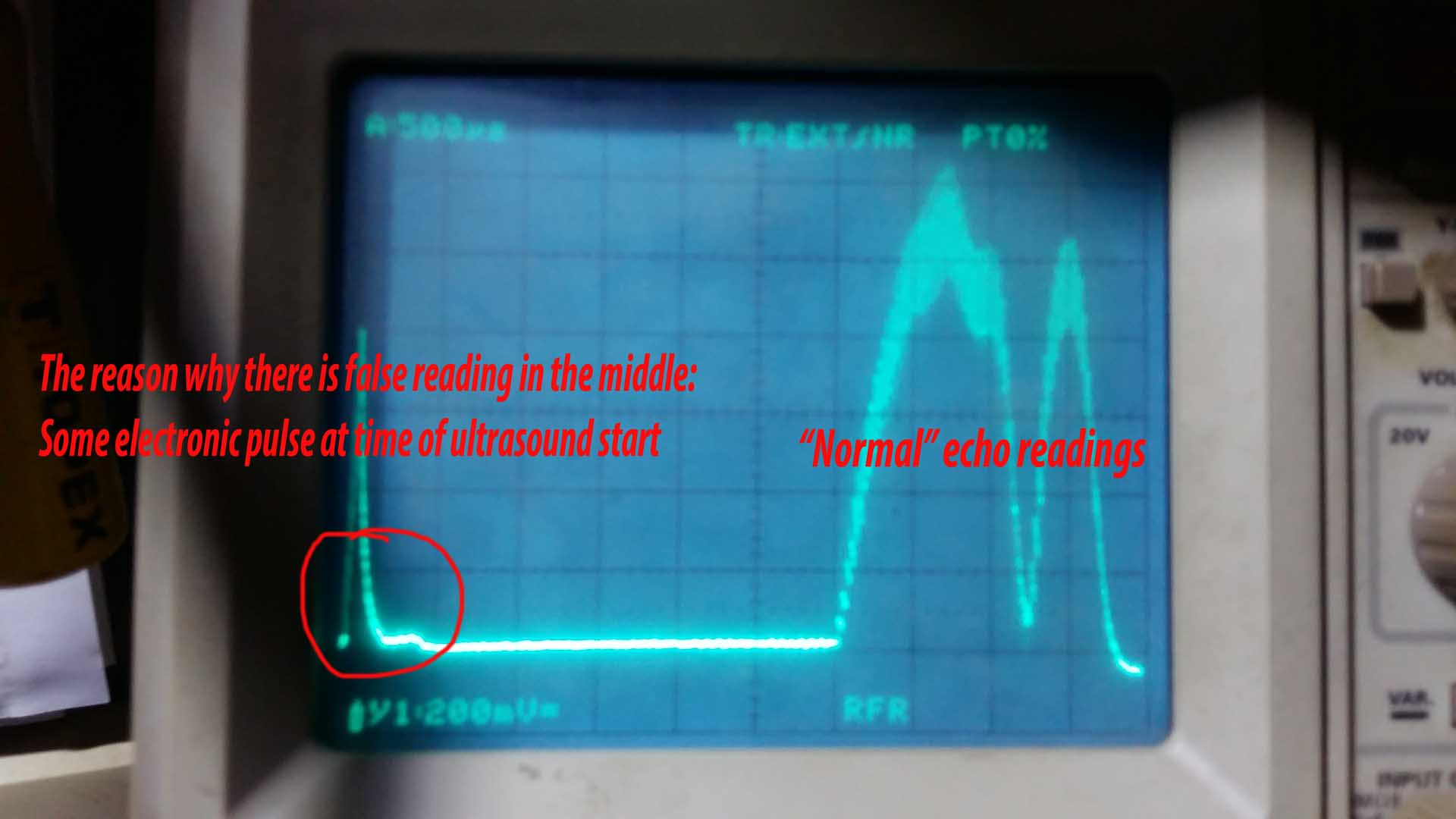# Ultrasonic Radar – Arduino project

This ultrasonic radar, or ultrasonic scanner – may be used for fun and learning Arduino code  (sketch) by changing parameters. As a bonus, there is Java program which uses Java code: Processing for various tasks and visualization tool. Very powerful tool (okay complicated at first, but anyone can learn how to make own code). Install it on your computer, and you may learn how to visualize data collected by various sensors attached to Arduino, or use it for whatsoever you want. It is free program, and working perfectly. An example of screenshot of Processing visualization is here:

## Ultrasonogram:Okay, image is not as good as Medical Ultrasound Scanner, but price is not as high as well (read: very, very expensive). 😀

Simple to make

This ultrasonic radar, in our case – image we get on the screen, we may call it “Sonogram”, or “Ultrasonogram” because it is more like medical ultrasonic scanner than military radar. More on wiki about this Sonogram. I am interested to get as much as possible points from the reflected ultrasonic waves at 41 kHz from HC-SR04 ultrasonic distance sensor. But, instead using “echo” pin, we will use analog output from amplified ultrasonic microphone. Milan-CAD:Also, one detail of simple attachment between sensor and servo:Just dab of hot-glue and job is done. If you change mind, you can “salvage” this servo for other experiments when ultrasound become boring (not so soon, I know myself). 😀

## Not the best artist on drawing

Out there are many programs for drawing diagrams, but all are complicated and/or confusing. Spending lot of time figuring out how it works is not the pleasant experience. If someone has good skill to making better drawing, feel free to draw it and back to me, or publish as your own drawing. I will not ask for any copyright rights on drawing, but I will insist on holding title of “original idea”. 😀

## Arduino Ultrasonic Multi-Point Radar sketch (old, as is in the video above):

```// Original idea by Milan Karakaš, https://wildlab.org (C)2016
// Made wild idea to use analog echo reading - NOT echo pin
// from HC-SR04 ultrasonic module, just solder one wire at
// collector of the transistor as is shown on my website:
// This sketch "burn" onto your favorite Arduino (uno, nano....)
// and enjoy in experimenting with ultrasound "Sonograms". :o)
// Pay attention to Serial.begin(115200) because there are lot
// of data sending in short period, so both: Arduino board and
// Processing program on your computer can communicate without
// los of data. Processing you can find here: https://processing.org/
// And your work will be published as "Showcase" for other people
// as an example. Later I will figure out how to reward you. Thanks.

#include <Servo.h>

#define SERVO_PWM_PIN 10
#define ANGLE_BOUNDS 80
#define ANGLE_STEP 1

int angle = 0;
int dir = 1;
int i,j;
float strength; //40 analog readings send to your computer for "Processing" program
Servo myservo;
int analog_pin = A0; //analog pin A0 on arduino nano, uno, mega....

void setup()
{
pinMode(2,OUTPUT);
Serial.begin(115200);
myservo.attach(SERVO_PWM_PIN);//later will be attached/detached, but once should start for first time
}

void loop()
{
getValues();
Serial.print(-angle, DEC);      //first parameter send to your computer for Processing program
// Pay Attention! There is "-angle" because some servoes may do it in wrong direction. If servo and image
// on your computer does not match, put there "angle" instead "-angle". This will reverse servo direction
for (i=0;i<40;i++)              //will be good to use variable instead fixed value for number of reading points
{                              //for later experimenting with number of analog read echo points
Serial.print(",");           // all data separated by coma (',')
Serial.print(strength[i],0); // Atention! This is not for debugging - Processing program will use it
// for visualisation (reading from COMxx port)
}
Serial.println(); //not printing anything, just new row, and back to the first place
myservo.write(angle + ANGLE_BOUNDS); //servo should move one step
if (angle >= ANGLE_BOUNDS || angle <= -ANGLE_BOUNDS)
{
dir = -dir;
}
angle += (dir*ANGLE_STEP);
delay(100); //alow some time for +5V to settle down, because servo can draw some current and make analog read error
}

int getValues() //function which read analog echo and return array of analog values
{
myservo.detach(); //very important! this command disable PWM - which make interference during analog read
delay(2); //wait additionally 2 mS for RF noise to settle down (first few centimeters)
digitalWrite(2,1); //this triggers sensor
delayMicroseconds(1); //although it is set to 1 uS, it gives actually 4 uS, which is sufficient to trigger HC-SR04 ultrasound ...
digitalWrite(2,0);//this is end of triggering sensor
delay(2);//lets avoid crosstalk between emitter and receiver in first millisecond or two
for (j=0;j<40;j++) //lets make array of j elements
{
int str= ((analogRead(analog_pin)*5.00)/1024)*pow(i,2)*5; // "dynamic amplification" by exponent^2 of i *5
// Since amplitude of ultrasound fall with square of the distance,
// "pow(i,2)" is actually i^2 (i squared). You may experiment with
// additional amplification by factor of 5 in example above
// too much, and noise will be visible, too low, and reflectin will be
// weak, yet maybe better "gray scale" than too high values
strength[j]=constrain(str,0,255); //since "Processing" program will use max values between 0 and 255, lets make some limits
}
myservo.attach(SERVO_PWM_PIN); //now let servo PWM signal to continue for next step before back into main loop
return strength; // returning array of analog read echo values, you may experiment with this number of points.
}                      // Just remember to change sketch on Processing so that number of this readings are correct
// Data send to your computer is angle + this number of samples. In Processing use array of 41 numbers
// First number will be from -80 to 80, which indicates angle, and the rest of numbers ranging fom 0 to 255
// representing amplitude of the echoed ultrasound.
```

## Processing sketch (old one; as in the video above):

```// Original idea by Milan Karakaš, 2016, https://wildlab.org
// Revision 0.99 - sorry, but not yet cleaned for not used parts
// some old stuff remained, because had hard time to figure out
// how to work in Processing. Many notes are in Croatian.
// Thanks for understanding.

import processing.serial.*;

int SIDE_LENGTH = 1000;
int ANGLE_BOUNDS = 80;
int ANGLE_STEP = 2;
int HISTORY_SIZE = 10;
int POINTS_HISTORY_SIZE = 500;
int MAX_DISTANCE = 100;

int angle;
int distance;
int[] echoes = new int; //možda array treba samo "int[] echoes"???
//Echo[] echoes; //možda bi ovo bio pravilniji array?! treba samo dodati to u class dolje

float x, y;

float[] historyX, historyY;
Point[] points; //ovo je primjer više točaka, no samo jedna je "prošla" kroz funkciju (angle, ->distance)

int centerX, centerY;

String comPortString;
Serial myPort;

void setup() {
size(1024, 600, P2D);
noStroke();
//smooth();
rectMode(CENTER);
background(0);
centerX = width / 2;
centerY = height;
angle = 0;

historyX = new float[HISTORY_SIZE];
historyY = new float[HISTORY_SIZE];
points = new Point[POINTS_HISTORY_SIZE];

myPort = new Serial(this, Serial.list(), 115200);
myPort.bufferUntil('\n'); // Trigger a SerialEvent on new line
}

void draw() //ovo je totalno okay, ne diraj
{
//background(0);
drawFoundObjects(angle, echoes); //bilo je: drawFoundObjects(angle, distance);
//  drawRadarLine(angle); //mislim da je ovo nepromijenjeno, no... dali to briše stare točke?
}

{
float px = centerX + x;
float py = centerY - y;
historyX = px;
historyY = py;
for (int i=0; i<HISTORY_SIZE; i++)
{
stroke(50, 150, 50, 255 - (25*i));
line(centerX, centerY, historyX[i], historyY[i]);
}
shiftHistoryArray();
}

void drawFoundObjects(int angle, int echoes[]) //bilo je: void drawFoundObjects(int angle, int distance)
{
int n;
//   int distance =500; // mimicking, imitiram kao da je odjek na "200" nečega
//ovdje je bila formula za test, maknuo dolje jer je nestala ako je broj veći od 0
for ( n=1;n<40;n++)
{
float distance = n*12.5;
if (distance > 0)
{
int px = (int)(centerX + x);
int py = (int)(centerY - y);
points = new Point(px, py); //bilo je: points = new Point(px, py);
} else
{
points = new Point(0, 0);
}
for (int i=0; i<POINTS_HISTORY_SIZE; i++) //mislim da je ovo definitivno jeba koja briše stare točke
{
Point point = points[i];//bilo je: Point point = points[i];
if (point != null)
{
int x = point.x;
int y = point.y;
if (x==0 && y==0) continue;
//int colorAlfa = (int)map(i, 0, POINTS_HISTORY_SIZE, 20, 0); //ovo je izgleda dio koji "briše" stare točke, treba maknuti

//  int size = (int)map(i, 0, POINTS_HISTORY_SIZE, 30, 5);
fill(echoes[n]); //ovdje bi trebao "sjesti" paremetar o jačini odjeka umjesto "255, ->15": fill(50, 150, 50, colorAlfa);
//ali colorAlfa "pojačava" stari trag. Ako se to ne želi, onda treba raditi bez alfe i "modulirati" prvi broj
noStroke();
ellipse(x, y, 15, 15); //maknuti smanjivanje točaka: ellipse(x, y, size, size);
/* temporary comfirmation that "echoes[]" are passing through void. YES!!!! SUCCESS!!!
for (int n=1; n<40; n++)
{
text("brojevi: " +  echoes[n],10,17*n);
println("br: " + echoes[n]); //ovo radi, samo sada treba "vratiti" vrijednosti za iscrtavanje točaka
//ispisivalo je dolje korektne vrijednosti, samo da nađem ...
} */
}
}

// shiftPointsArray();
}}

{
stroke(100);
noFill();

// part of the circle distance from the center
for (int i = 0; i <= (SIDE_LENGTH / 100); i++) {
}

// angle indicators
for (int i = 0; i <= (ANGLE_BOUNDS*2/20); i++) {
float angle = -ANGLE_BOUNDS + i * 20;
}
}

void shiftHistoryArray() {

for (int i = HISTORY_SIZE; i > 1; i--) {

historyX[i-1] = historyX[i-2];
historyY[i-1] = historyY[i-2];
}
}

void shiftPointsArray(){}//ovo treba izbaciti ZAUVIJEK!!!
/*{
for (int i = POINTS_HISTORY_SIZE; i > 1; i--) {

Point oldPoint = points[i-2];
if (oldPoint != null) {

Point point = new Point(oldPoint.x, oldPoint.y);
points[i-1] = point; //ovo nedostaje gore?
}
}
}*/

void serialEvent(Serial cPort)
{

if (comPortString != null)
{

comPortString=trim(comPortString);
String[] values = split(comPortString, ',');
try
{
angle = Integer.parseInt(values); //ovo razjebati
//distance = int(map(Integer.parseInt(values), 1, MAX_DISTANCE, 1, radius)); //ovo treba razjebati i preopraviti
//ovdje ću početi razjebavati
//println("brojevi su:");

for (int n=1; n<40; n++) //nikako "0", jer je to kut - sve ostalo su analogne vrijednosti od 0-255
{
echoes[n]= Integer.parseInt(values[n]);
//textSize(12);
//fill(255);
//text("brojevi: " +  echoes[n],10,17*n);
//println("br: " + echoes[n]); //ovo radi, samo sada treba "vratiti" vrijednosti za iscrtavanje točaka
//ispisivalo je dolje korektne vrijednosti, samo da nađem ...
}
}
catch (Exception e) {}
}

}

class Point {
int x, y;

Point(int xPos, int yPos) {
x = xPos;
y = yPos;
}

int getX() {
return x;
}

int getY() {
return y;
}
}
```

## New (and better) Arduino sketch for ultrasonic radar, some parts optimized:

```// Original idea by Milan Karakaš, https://wildlab.org (C)2016
// Made wild idea to use analog echo reading - NOT echo pin
// from HC-SR04 ultrasonic module, just solder one wire at
// collector of the transistor as is shown on my website:
// This sketch "burn" onto your favorite Arduino (uno, nano....)
// and enjoy in experimenting with ultrasound "Sonograms". :o)
// Pay attention to Serial.begin(115200) because there are lot
// of data sending in short period, so both: Arduino board and
// Processing program on your computer can communicate without
// los of data. Processing you can find here: https://processing.org/
// And your work will be published as "Showcase" for other people
// as an example. Later I will figure out how to reward you. Thanks.

#include <Servo.h>

#define SERVO_PWM_PIN 10
#define ANGLE_BOUNDS 80
#define ANGLE_STEP 1

int angle = 0;
int dir = 1;
int i,j;
float strength; //40 analog readings send to your computer for "Processing" program
Servo myservo;
int analog_pin = A0; //analog pin A0 on arduino nano, uno, mega....

void setup()
{
pinMode(2,OUTPUT);
Serial.begin(115200);
//Serial.begin(250000);
myservo.attach(SERVO_PWM_PIN);//later will be attached/detached, but once should start for first time
}

void loop()
{
getValues();
Serial.print(-angle, DEC);      //first parameter send to your computer for Processing program
// Pay Attention! There is "-angle" because some servoes may do it in wrong direction. If servo and image
// on your computer does not match, put there "angle" instead "-angle". This will reverse servo direction
for (i=0;i<80;i++)              //will be good to use variable instead fixed value for number of reading points
{                              //for later experimenting with number of analog read echo points
Serial.print(",");           // all data separated by coma (',')
Serial.print(strength[i],0); // Atention! This is not for debugging - Processing program will use it
// for visualisation (reading from COMxx port)
}
Serial.println(); //not printing anything, just new row, and back to the first place
myservo.write(angle + ANGLE_BOUNDS); //servo should move one step
if (angle >= ANGLE_BOUNDS || angle <= -ANGLE_BOUNDS)
{
dir = -dir;
}
angle += (dir*ANGLE_STEP);
delay(10); // alow some time for +5V to settle down, because servo can draw some current and make analog read error
// DO NOT go below 10 mS - this value is experimentally get by oscilloscope, else strange "rays" may occur on radar
}

int getValues() //function which read analog echo and return array of analog values
{
myservo.detach(); //very important! this command disable PWM - which make interference during analog read
delay(2); //wait additionally 2 mS for RF noise to settle down (first few centimeters)
digitalWrite(2,1); //this triggers sensor
delayMicroseconds(1); //although it is set to 1 uS, it gives actually 4 uS, which is sufficient to trigger HC-SR04 ultrasound ...
digitalWrite(2,0);//this is end of triggering sensor
// delay(1);//lets avoid crosstalk between emitter and receiver in first millisecond or two
// NO! let it be: if this delay is added, then whole screen is wrong - missing some components and distorted...
// As a result of avoiding this delay, "origin" of the radar pulses will be with some false reading, but not a problem
for (j=0;j<80;j++) //lets make array of j elements
{
// int str= ((analogRead(analog_pin)*5.00)/1024)*pow(i,2);   // too long to execute, also not using constrain below
strength[j]=(analogRead(analog_pin)*0.0025)*pow(i,2); // "dynamic amplification" by exponent^2 of i *5
// Since amplitude of ultrasound fall with square of the distance,
// "pow(i,2)" is actually i^2 (i squared). You may experiment with
// additional amplification by factor of 5 in example above
// too much, and noise will be visible, too low, and reflectin will be
// weak, yet maybe better "gray scale" than too high values
//strength[j]=constrain(str,0,255); //since "Processing" program will use max values between 0 and 255, lets make some limits
//but disabled because it "stills" some valuable mathematic time of the MCU
}
myservo.attach(SERVO_PWM_PIN); //now let servo PWM signal to continue for next step before back into main loop
return strength; // returning array of analog read echo values, you may experiment with this number of points.
}                      // Just remember to change sketch on Processing so that number of this readings are correct
// Data send to your computer is angle + this number of samples. In Processing use array of 41 numbers
// First number will be from -80 to 80, which indicates angle, and the rest of numbers ranging fom 0 to 255
// representing amplitude of the echoed ultrasound.
```

## Processing sketch for ultrasonic radar, cleaned (to some extent), and added more points (now 80 instead 40):

```// Original idea by Milan Karakaš, 2016, https://wildlab.org
// Revision 1.01 - sorry, but not yet cleaned for not used parts
// some old stuff remained, because had hard time to figure out
// how to work in Processing. Thanks for understanding.
// New big screen size; 1920 x 1024 for those who have HD screen
// Or, down below should be math slightly changed

import processing.serial.*;

int SIDE_LENGTH = 1000;
int ANGLE_BOUNDS = 80;
int ANGLE_STEP = 2;
int HISTORY_SIZE = 10;
int POINTS_HISTORY_SIZE = 500;
int MAX_DISTANCE = 100;

int angle;
int distance;
int[] echoes = new int; // Now with more points; 80 instead old 40

float x, y;

float[] historyX, historyY;
Point[] points; //ovo je primjer više točaka, no samo jedna je "prošla" kroz funkciju (angle, ->distance)

int centerX, centerY;

String comPortString;
Serial myPort;

void setup() {
size(1920, 1024, P2D);// was 1024,600 - for 80 points it is better to have bigger screen
noStroke();
//smooth();
rectMode(CENTER);
background(0);
centerX = width / 2;
centerY = height;
angle = 0;

historyX = new float[HISTORY_SIZE];
historyY = new float[HISTORY_SIZE];
points = new Point[POINTS_HISTORY_SIZE];

myPort = new Serial(this, Serial.list(), 115200);
//  myPort = new Serial(this, Serial.list(), 250000); //just tested in debugging process, ignore
myPort.bufferUntil('\n'); // Trigger a SerialEvent on new line
}

void draw() //ovo je totalno okay, ne diraj
{
drawFoundObjects(angle, echoes); //was: drawFoundObjects(angle, distance);
}

void drawFoundObjects(int angle, int echoes[]) //was: void drawFoundObjects(int angle, int distance)
{
int n;
for ( n=1;n<80;n++)
{
//float distance = n*6.25; //for "small screen, or one with only 40 points
float distance = n*12.5;// "zoom" options   -  much bigger screen
if (distance > 0)
{
int px = (int)(centerX + x);
int py = (int)(centerY - y);
points = new Point(px, py); //was: points = new Point(px, py);
} else
{
points = new Point(0, 0);
}
for (int i=0; i<POINTS_HISTORY_SIZE; i++) //not resolved yet whether it needs to be here or not...
{
Point point = points[i];//was: Point point = points[i];
if (point != null)
{
int x = point.x;
int y = point.y;
if (x==0 && y==0) continue;

// NEW, "green" look of the screen :D
fill(20,echoes[n],40); //or "fill(echoes[n])", or "fill(255,echoes[n]", or "fill(echoes[n],echoes[n])" - gives various graphic result
//first number in "fill(number,alpha) is brightnes of elypse, and second is "alpha" whatsoever, different effect
noStroke();
ellipse(x, y, 7, 7); //variation on 15,15 if necessary: ellipse(x, y, size, size); //various spot sizes...
}
}

}
}

{
stroke(100);
noFill();

// part of the circle distance from the center
for (int i = 0; i <= (SIDE_LENGTH / 100); i++) {
}

// angle indicators
for (int i = 0; i <= (ANGLE_BOUNDS*2/20); i++) {
float angle = -ANGLE_BOUNDS + i * 20;
}
}

void serialEvent(Serial cPort)
{

if (comPortString != null)
{

comPortString=trim(comPortString);
String[] values = split(comPortString, ',');
try
{
angle = Integer.parseInt(values); //just first element of array is angle, everything else are echoes analog values
for (int n=1; n<80; n++) //new 80 elements instead 40
{
echoes[n]= Integer.parseInt(values[n]);
}
}
catch (Exception e) {}
}

}

class Point {
int x, y;

Point(int xPos, int yPos) {
x = xPos;
y = yPos;
}

int getX() {
return x;
}

int getY() {
return y;
}
}
```

## New, better look; 80 points, and “Military green” colors 😀## New video  example of improved program on Arduino and Processing:

Only one part is wrong due to electronic noise: “origin”, or center of rotation is always present. Tried “delay(2)”, or two milliseconds in the loop for reading analog data, but this gives distortion and whole screen looks like it will “sink” into this central part – because missing few point rows. Now, it looks okay. Maybe there are some room for further improvements, will see.

## The reason of the central “ghost readings”:Just after ultrasonic frequencies are off, amplifier still has some spike, which looks strange on the radar screen. But, this is not such big problem. Instead “blanking-out” at Arduino end, it is maybe better to do it at Processing end on your computer. For me, this is not issue at all.